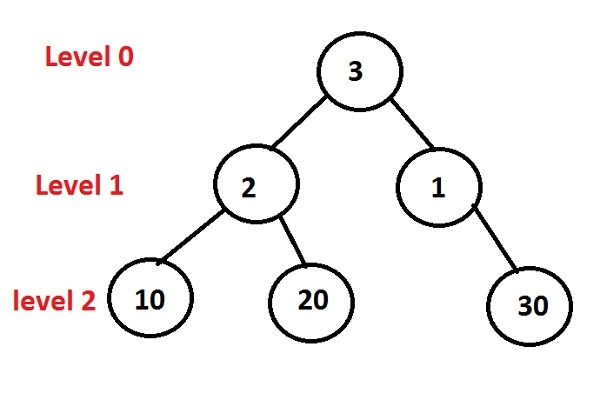# Print level order traversal line by line in C++ Programming.

Given the binary tree, the function must find out the level order traversal of a tree line by line.

Level order traversal: Left Root Right, which means firstly print left child of a node than the value of a root and then go to the right child but here we have to do line by line which will start from left and will end at the right node of a given binary tree.The binary tree given above will generate the following output −

Level 0: 3
Level 1: 2 1
Level 2: 10 20 30

## Algorithm

START
Step 1 -> create a structure of a node as
struct node
struct node *left, *right
int data
End
Step 2 -> function to create a node
node* newnode(int data)
node *temp = new node
temp->data = data
temp->left = temp->right= NULL
return temp
step 3 -> function for inorder traversal
void levelorder(node *root)
IF root = NULL
Return
End
queue<node *> que
que.push(root)
Loop While que.empty() = false
int count = que.size()
Loop While count > 0
node *node = que.front()
print node->data
que.pop()
IF node->left != NULL
que.push(node->left)
End
IF node->right != NULL
que.push(node->right)
End
Decrement count by 1
End
End
Step 4 -> In main() function
Create tree using node *root = newnode(3)
Call levelorder(root)
STOP

## Example

Live Demo

#include <iostream>
#include <queue>
using namespace std;
//it will create a node structure
struct node{
struct node *left;
int data;
struct node *right;
};
void levelorder(node *root){
if (root == NULL)
return;
queue<node *> que;
que.push(root);
while (que.empty() == false){
int count = que.size();
while (count > 0){
node *node = que.front();
cout << node->data << " ";
que.pop();
if (node->left != NULL)
que.push(node->left);
if (node->right != NULL)
que.push(node->right);
count--;
}
}
}
//it will create a new node
node* newnode(int data){
node *temp = new node;
temp->data = data;
temp->left = NULL;
temp->right = NULL;
return temp;
}
int main(){
// it will generate the binary tree
node *root = newnode(3);
root->left = newnode(2);
root->right = newnode(1);
root->left->left = newnode(10);
root->left->right = newnode(20);
root->right->right = newnode(30);
levelorder(root);
return 0;
}

## Output

if we run the above program then it will generate the following output

3 2 1 10 20 30